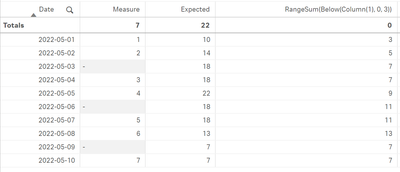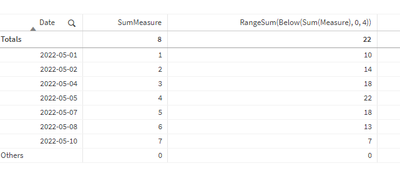# App Development

Announcements
cancel
Showing results for
Did you mean:Contributor II

## Sum of next N non null values

Hi,

I have a table chart like this:I'm trying to get the sum of the next 4 non null values (including current value). So I want to start by looking at the current row, and if it's not null gather it. Continue to next row and do the same. When 4 non null values have been collected, I want to sum them. And it needs to happen in the UI/Chart, not in the script.

I've only been able to use Below, but then I need to set a larger window and risk summing more than the next 4 values. If I set it as RangeSum(Below(Column(1), 0 , 4)) then I risk summing fewer values than intended.

I tried using FirstSortedValue and put the null values at the bottom of the sorting, but I think it works by looking at the internal table and not the chart.

Labels (1)
• ### General Question

2 RepliesEmployee

If you exclude the nulls from your chart (in Date column use Limitation > Exact value >0)
and extend range to 4, you get your result ..Contributor II
Author

I'm sorry for being unclear. This is not an option, all rows must exist.

The solution I have so far is this:

``````Let _var_PBC_MinSignalWindow    = 8;
Let _var_PBC_MaxSignalWindow    = \$(_var_PBC_MinSignalWindow)*2;

For i = 0 to \$(_var_PBC_MaxSignalWindow)

Let _var_PBC_SignalWindow       = \$(_var_PBC_MinSignalWindow) + \$(i);
Let _var_PBC_SignalBelow        = 'Below(Column(1), 0, \$(_var_PBC_SignalWindow))';
Let _var_PBC_SignalBelowCheck   = 'RangeNumericCount(\$(_var_PBC_SignalBelow)) = \$(_var_PBC_MinSignalWindow)';
Let _var_PBC_RangeMin_Formula   = 'If(\$(_var_PBC_SignalBelowCheck), RangeMin(\$(_var_PBC_SignalBelow)))';
Let _var_PBC_RangeMax_Formula   = 'If(\$(_var_PBC_SignalBelowCheck), RangeMax(\$(_var_PBC_SignalBelow)))';

Switch i
Case 0
Let _var_PBC_RangeMin   = '\$(_var_PBC_RangeMin_Formula)';
Let _var_PBC_RangeMax   = '\$(_var_PBC_RangeMax_Formula)';

Default
Let _var_PBC_RangeMin   = '\$(_var_PBC_RangeMin), \$(_var_PBC_RangeMin_Formula)';
Let _var_PBC_RangeMax   = '\$(_var_PBC_RangeMax), \$(_var_PBC_RangeMax_Formula)';

End Switch

Let _var_PBC_SignalWindow       = Null();
Let _var_PBC_SignalBelow        = Null();
Let _var_PBC_SignalBelowCheck   = Null();
Let _var_PBC_RangeMin_Formula   = Null();
Let _var_PBC_RangeMax_Formula   = Null();

Next i

Let i = Null();

Set _var_PBC_RangeMin = Alt(\$(_var_PBC_RangeMin));
Set _var_PBC_RangeMax = Alt(\$(_var_PBC_RangeMax));``````

So basically I do a RangeNumericCount for the range N, if that matches N, then proceed.

Else, check N+1, N+2, N+3... N*2